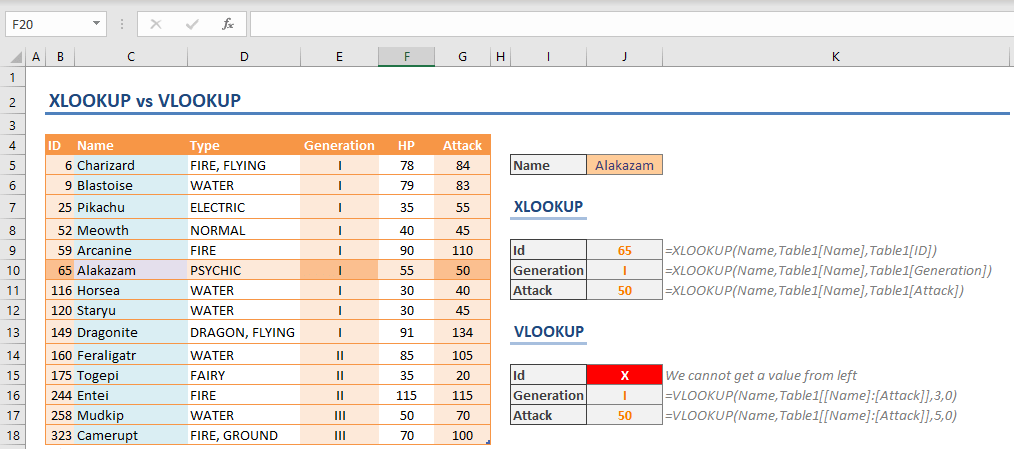In this article, we are going to show you the differences between XLOOKUP and VLOOKUP functions.

Please note that the XLOOKUP function is currently only accessible for Microsoft 365 subscribers.

XLOOKUP(lookup_value, lookup_array, return_array, [if_not_found], [match_mode], [search_mode])

## Lookup to the left

The first difference between XLOOKUP and VLOOKUP functions is the biggest limitation of the VLOOKUP: You can only search for a value in the left-most column of a table and retrieve a value from the columns to its right. A common workaround is using the INDEX-MATCH combination. With XLOOKUP you can get a value from any side of the search value.

Instead of selecting the entire range, the XLOOKUP requires 2 ranges:

• lookup_array: The array or range where you want to look up the value
• return_array: The array or range where you want to return a value from

As a result, you can select search and lookup ranges separately. In the following example, the formula searches for “Alakazam” in the Name column (Table13[Name]) and returns the ID value (Table13[ID]) matching the name.## No column index

The col_index_num argument which determines the index of the return column for VLOOKUP. The XLOOKUP function, however, doesn't need a parameter for counting columns.

Column index number can be tricky to determine for large tables. Furthermore, deleting a column can change indexes of each column after the deleted one. For example, if you delete the 3rd column from a 5-column table, the previous 4th and 5th columns will become 3rd and 4th.

You can see the argument differences between XLOOKUP and VLOOKUP below.## Default match type

The default match type is “exact match” in XLOOKUP. VLOOKUP requires this to be defined with the range_lookup argument.

The XLOOKUP contains a similar argument named match_type as well. A 0 value here will make the function work like VLOOKUP.

## Approximate match types

VLOOKUP’s range_lookup argument can have 2 values:

• 1: returns the value corresponding to the next smaller value if the search value does not exist
• 0: returns the corresponding value only if the search value exists

The XLOOKUP’s match_type has the additional options below:

• -1: returns the value corresponding to the next smaller if the search value does not exist
• 0: returns the corresponding value only if the search value exists
• 1: returns the value corresponding to the next greater if the search value does not exist
• 2: wildcard support

Thus, range_lookup = 1 works the same way as match_type = -1. VLOOKUP has wildcard support, and with the XLOOKUP, you can choose to include this as well.

Another improvement on approximate search is that you do not need to sort your data beforehand. Let's take the example below. Both the XLOOKUP and the VLOOKUP are doing an approximate search. However, the VLOOKUP returns #N/A because the data is not sorted.Thanks to the XLOOKUP’s if_not_found argument, you do not need to use other functions like IFERROR or IFNA to handle scenarios where no matches are found.## Summary and tips

• You can make right-to-left lookups with XLOOKUP.
• Adding or removing columns do not affect XLOOKUP.
• Approximate search can work both ways.
• No need to sort data for approximate search.
• You can determine search direction.
• The XLOOKUP doesn’t need entire table to be referenced - only search and return columns.

Excel supports VLOOKUP, HLOOKUP, and LOOKUP functions for backwards compatibility, but the new lookup formula can replace them all, and offers more functionality.